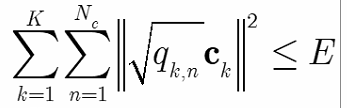# How to solve this problem {real affine}.*{convex}

I have a constraint as followswhere qk,n and ck are variables

but the cvx occurs error

{real affine}.*{convex}

if `q` and `c` are both variables, that is non-convex. If only one of them were a variable, it would be possible to formulate in CVX.

Sorry, why it is non-convex?